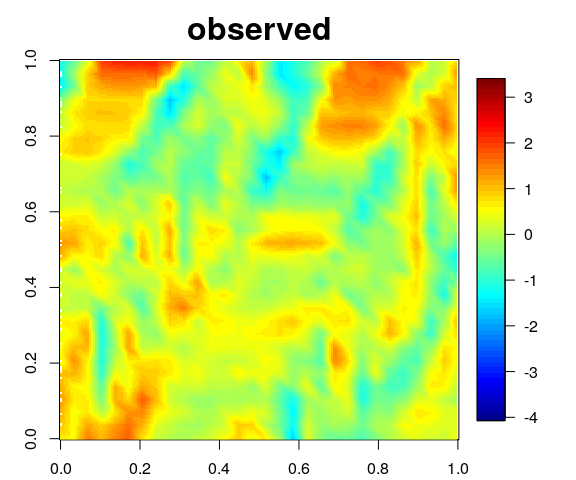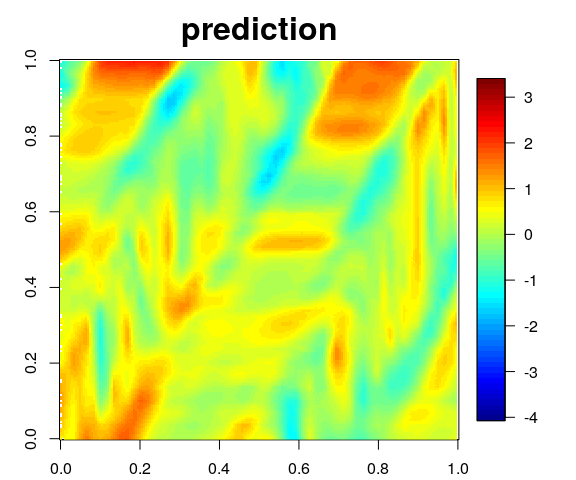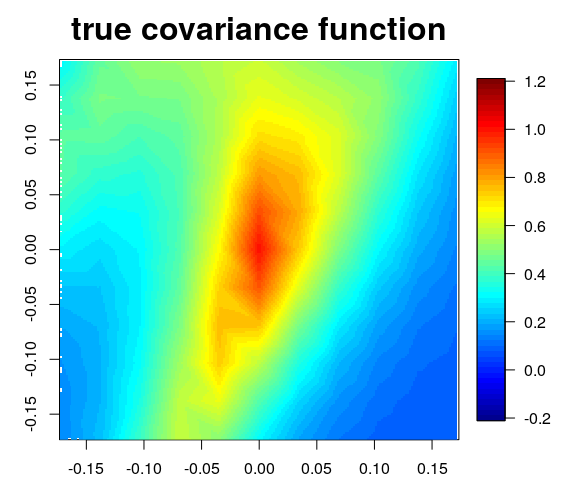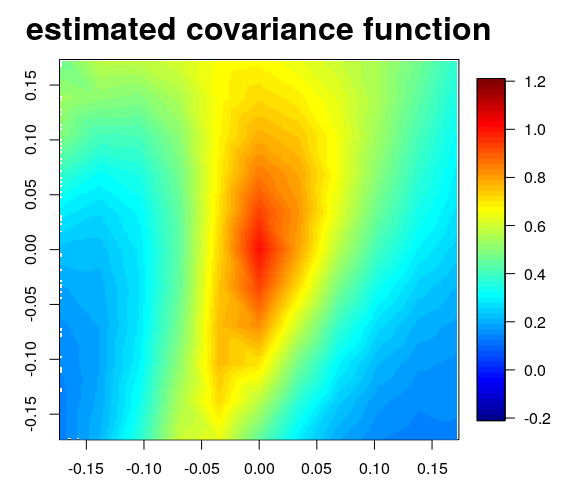# NSGPR example

library(GPFDA)
require(MASS)

## Simulating data from a GP with nonstationary and nonseparable covariance structure

In this example, we simulate data from a GP whose covariance structure has a spatially-varying anisotropy matrix.

We simulate $$10$$ independent realizations of $$X(\boldsymbol{{t}}), \ \boldsymbol{{t}} = (s_1, s_2)^\top$$, from the following model: $\begin{equation} X(\boldsymbol{{t}}) = f(\boldsymbol{{t}}) + \varepsilon(\boldsymbol{{t}}), \quad \varepsilon(\boldsymbol{{t}}) \sim N(0, \sigma_\varepsilon^2), \quad \text{ where } \boldsymbol{{t}} \in \mathcal{T} \subset \left[0,1\right]^2. \end{equation}$ We assume that $$f$$ is a zero-mean GP with a nonseparable and nonstationary covariance function given by \begin{align}\label{NScovfun} \text{Cov}\big[ f(\boldsymbol{{t}}),f(\boldsymbol{{t}}') \big] = \sigma(\boldsymbol{{t}}) \sigma(\boldsymbol{{t}}') | \boldsymbol{{A}}(\boldsymbol{{t}}) | ^{-1/4} | \boldsymbol{{A}}(\boldsymbol{{t}}') | ^{-1/4} \times \bigg| \frac{\boldsymbol{{A}}^{-1}(\boldsymbol{{t}}) + \boldsymbol{{A}}^{-1}(\boldsymbol{{t}}')}{2} \bigg| ^{-1/2} g\Big( \sqrt{Q_{\boldsymbol{{t}}\boldsymbol{{t}}'}}\Big), \end{align} where $$g(\cdot)$$ is the exponential correlation function, $$\sigma(\boldsymbol{{t}})=1$$ and $$\sigma_\varepsilon^2$$ is negligible.

The elements of the varying anisotropy matrix $$\boldsymbol{{A}}(s_1,s_2)$$ are: \begin{align} & \boldsymbol{{A}}_{11}(s_1,s_2) = \exp\big(6 \cos(10 s_1 - 5 s_2)\big), \\ & \boldsymbol{{A}}_{22}(s_1,s_2) = \exp\big(\sin(6 s_1^3) + \cos(6 s_2^4)\big),\\ & \boldsymbol{{A}}_{12}(s_1,s_2) = \{\boldsymbol{{A}}_{11}(s_1,s_2)\boldsymbol{{A}}_{22}(s_1,s_2)\}^{1/2}\rho_{12}(s_1,s_2), \\ & \rho_{12}(s_1,s_2) = \tanh\big((s_1^2+s_2^2)/2\big). \end{align}

set.seed(123)
n1 <- 30 # sample size for input coordinate 1
n2 <- 30 # sample size for input coordinate 2
n <- n1*n2  # total sample size

# Creating evenly spaced spatial coordinates
input <- list()
input[] <- seq(0,1,length.out = n1)
input[] <- seq(0,1,length.out = n2)
inputMat <- as.matrix(expand.grid(input)) # inputs in matrix form

# Creating the varying anisotropy matrix with spatially-varying parameters
A11 <- function(s1,s2){
exp(6*cos(10*s1 - 5*s2))
}
A22 <- function(s1,s2){
exp(sin(6*s1^3) + cos(6*s2^4))
}
A12 <- function(s1,s2){
sqrt(A11(s1,s2)*A22(s1,s2))*tanh((s1^2+s2^2)/2)
}
A_List <- list()
R12_vec <- rep(NA, n)
for(i in 1:n){
s1 <- inputMat[i,1]
s2 <- inputMat[i,2]
A_i11 <- A11(s1=s1, s2=s2)
A_i22 <- A22(s1=s1, s2=s2)
A_i12 <- A12(s1=s1, s2=s2)
A_i <- matrix(NA, 2, 2)
A_i[1,1] <- A_i11
A_i[2,2] <- A_i22
A_i[1,2] <- A_i12
A_i[2,1] <- A_i12
A_List[[i]] <- A_i
R12_vec[i] <- A_i12/sqrt(A_i11*A_i22)
}

# Constructing the (n x n) covariance matrix K
ScaleDistMats <- calcScaleDistMats(A_List=A_List, coords=inputMat)
Scale.mat <- ScaleDistMats$Scale.mat Dist.mat <- ScaleDistMats$Dist.mat

corrModel <- "pow.ex"
gamma <- 1
K <- Scale.mat*unscaledCorr(Dist.mat=Dist.mat, corrModel=corrModel, gamma=gamma)
diag(K) <- diag(K) + 1e-8

# Generate response surfaces
meanFunction <- rep(0, n)
nrep <- 10
response <- t(mvtnorm::rmvnorm(nrep, meanFunction, K))

## Estimating NSGP covariance function

The estimation of the NSGP covariance function is done by using the nsgpr function.

We want a nonseparable covariance structure (sepCov=F), assuming the process has unit variance (unitSignalVariance=T) and a zero noise variance (zeroNoiseVariance=T).

The unconstrained parameters associated to the varying anisotropy matrix will be modeled via $$L=M=6$$ B-spline basis functions with evenly spaced knots. To allow the parameters of the anisotropy matrix to change along both directions $$s_1$$ and $$s_2$$, we set whichTau = c(T,T).

The estimated hyperparameters (B-spline coefficients) from the nsgpr function are saved in the object hp.

### NOT RUN

# fit <- nsgpr(response = response,
#               input = input,
#               corrModel = corrModel,
#               gamma = gamma,
#               whichTau = c(T,T),
#               absBounds = 8,
#               nBasis = 6,
#               nInitCandidates = 1000,
#               cyclic = c(F,F),
#               unitSignalVariance = T,
#               zeroNoiseVariance = T,
#               sepCov = F)

## Taking ML estimates of B-spline coefficients

# hp <- fit$MLEsts ### end NOT RUN hp <- c(5.60699, 1.14865, -6.61148, 8, -4.44439, -2.14159, 3.98823, 5.42213, -8, 6.91389, -0.17032, -6.29215, 0.48661, 8, -2.84537, -1.59173, 8, -2.55939, -8, -0.95508, 7.58644, -8, 4.86161, 8, -4.08238, -8, 8, -2.74033, -3.5963, 8, -1.30662, -8, 2.05985, 4.27012, -7.26238, 4.76814, 0.67189, 0.62838, 0.42846, 1.33653, -0.40139, 0.43309, -0.74954, 0.79752, -1.159, 3.31145, -0.87894, -0.99702, 1.72585, -0.29033, 2.41099, 0.46065, -0.95101, 2.0856, -1.39188, 0.57495, -0.39334, 3.24682, 1.09657, -1.90269, 1.5223, -2.87977, 1.54015, -3.89324, -1.67514, -0.18049, 3.93999, -1.50017, 1.18639, 2.16521, -6.93837, -1.88978, -2.06261, -0.80103, 3.17891, -3.68428, 1.64603, 0.58847, 0.86276, -2.38605, 3.99548, -0.52069, 1.33181, -0.10872, -0.43153, -4.91787, 2.56248, 1.90786, -5.382, 0.42587, 1.43742, 0.54047, -0.23679, 2.63721, -0.11159, 0.57184, -2.06124, 1.82977, -6.13951, 4.22915, -0.29822, -2.69144, -7.61238, 5.1746, -5.04487, 7.5953, 0.16564, -1.16696, 0, 0, 0, 0, 0, 0, 0, 0, 0, 0, 0, 0, 0, 0, 0, 0, 0, 0, 0, 0, 0, 0, 0, 0, 0, 0, 0, 0, 0, 0, 0, 0, 0, 0, 0, 0, -23.02585) ## Prediction Based on the estimated NSGP model, we want to make predictions at test input points: # Creating test input points n1test <- n1*2 n2test <- n2*2 inputNew <- list() inputNew[] <- seq(0,1,length.out = n1test) inputNew[] <- seq(0,1,length.out = n2test) inputMatTest <- as.matrix(expand.grid(inputNew[], inputNew[])) pred <- nsgprPredict(hp=hp, response=response, input=input, inputNew=inputNew, noiseFreePred=F, nBasis=6, corrModel=corrModel, gamma=gamma, cyclic=c(F,F), whichTau=c(T,T)) Below we plot the first realisation and then the corresponding predictions for the new test input locations: zlim <- range(c(pred$pred.mean, response))
plotImage(response = response, input = inputMat, realisation = 1,
n1 = n1, n2 = n2,
zlim = zlim, main = "observed")plotImage(response = pred$pred.mean, input = inputMatTest, realisation = 1, n1 = n1test, n2 = n2test, zlim = zlim, main = "prediction")## Plots of covariance functions To calculate the covariance matrix based on the hp estimates found above: FittedCovMat <- nsgpCovMat(hp=hp, input=input, corrModel=corrModel, gamma=gamma, nBasis=6, cyclic=c(F,F), whichTau=c(T,T), calcCov=T)$Cov

We will plot slices of the true and fitted covariance functions centred at specific spatial locations (the $$8$$th observed point of $$s_1$$ and the $$10$$th of $$s_2$$). The idea is to obtain image plots of $$k(s_1-0.24, s_2-0.31; 0.24, 0.31)$$.

# centre points for the covariance functions
input1cent <- input[]
input2cent <- input[]
# half-width
maxdist <- 0.2

zlim <- range(c(K, FittedCovMat)) + 0.2*c(-1,1)
centre_idx <- which(inputMat[,1]==input1cent & inputMat[,2]==input2cent)
other_idx <- which(inputMat[,1]>input1cent-maxdist &
inputMat[,1]<input1cent+maxdist &
inputMat[,2]>input2cent-maxdist &
inputMat[,2]<input2cent+maxdist)
other_idx_le <- length(other_idx)

# Locations of the slice:
sliceInputMat <- inputMat[other_idx,] - cbind(rep(input1cent, other_idx_le),
rep(input2cent, other_idx_le))

# Slice of the true covariance matrix
sliceCov <- K[centre_idx, other_idx]
nEach <- sqrt(length(sliceCov))
sliceTrueCov <- c(t(matrix(sliceCov, byrow=T, ncol=nEach)))

plotImage(response = sliceTrueCov, input = sliceInputMat,
n1 = nEach, n2 = nEach,
zlim = zlim, main = "true covariance function")# Slice of the estimated covariance matrix
sliceCov <- FittedCovMat[centre_idx, other_idx]
nEach <- sqrt(length(sliceCov))
sliceFittedCov <- c(t(matrix(sliceCov, byrow=T, ncol=nEach)))

plotImage(response = sliceFittedCov, input = sliceInputMat,
n1 = nEach, n2 = nEach,
zlim = zlim, main = "estimated covariance function")